# How to divide algebraic fractions

Want to find out how to divide algebraic fractions? We've provided a step-by-step guide of how to divide algebraic fractions to teach you everything you need to know.## Step 1: Find the reciprocal of the divisor

Find the reciprocal of the divisor, which is the fractions after the ÷ symbol. So, if we divide (9x^2)/24y by 5x/(8y^3 ), find the reciprocal of 5x/(8y^3 ) first.

## Step 2: Multiply the dividend by the reciprocal

Once the reciprocal of the divisor is determined, multiply the dividend by the reciprocal of the divisor. So, for our example, we multiply (9x^2)/24y by (8y^3)/5x .

## Step 3: Simplify the fractions

Look for common factors that can be cancelled to simplify the fractions.

## Step 4: Multiply remaining factors

Multiply the remaining factors to find the simplified quotient.

## Examples of how to divide algebraic fractions

Q1) Find the quotient of the following algebraic fractions.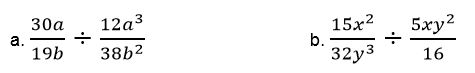Determine the reciprocal of the divisors (the algebraic fraction after ÷). Hence the following are the reciprocals of the divisor.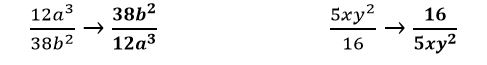Once we have the reciprocals of the divisors, multiply both dividend and reciprocal.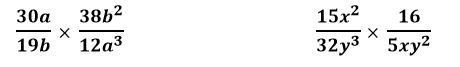Cancel out common factors shared by the numerator and denominator.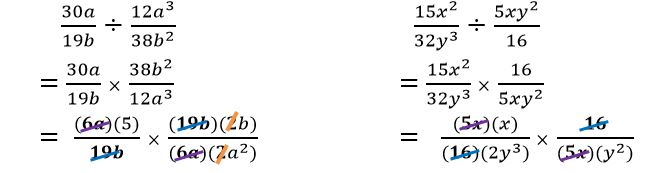Multiply the remaining the factors to find the quotients. Hence, we have: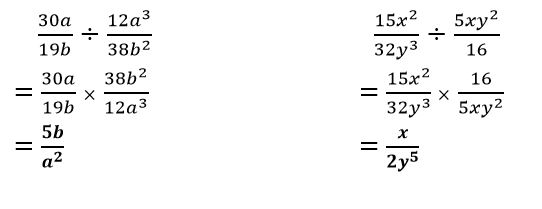#### Join today

The fastest way to practice

Unlock our complete testing platform and improve faster that ever.